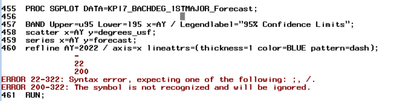## Add a vertical reference line to time series plot in sas

I try to add a vertical reference line to a time series plot to indicate the year where the forecast starts, but keep getting this error message in sas log. The variable AY has the datetime format Year4. The sas code works for the plot until I add the refline statement. Will appreciate any help with the refline statement! Thanks!``````PROC SGPLOT DATA=BACHDEG_Forecast;
BAND Upper=u95 Lower=l95 x=AY / Legendlabel="95% Confidence Limits";
scatter x=AY y=degrees_usf;
series x=AY y=forecast;
refline "AY=2022" / axis=x lineattrs=(thickness=1 color=BLUE pattern=dash);
RUN;``````
1 ACCEPTED SOLUTION

Accepted Solutions

## Re: Add a vertical reference line to time series plot in sas

@xliu1 wrote:

I try to add a vertical reference line to a time series plot to indicate the year where the forecast starts, but keep getting this error message in sas log. The variable AY has the datetime format Year4. The sas code works for the plot until I add the refline statement. Will appreciate any help with the refline statement! Thanks!``````PROC SGPLOT DATA=BACHDEG_Forecast;
BAND Upper=u95 Lower=l95 x=AY / Legendlabel="95% Confidence Limits";
scatter x=AY y=degrees_usf;
series x=AY y=forecast;
refline "AY=2022" / axis=x lineattrs=(thickness=1 color=BLUE pattern=dash);
RUN;``````

REFLINE wants either a VARIABLE or an explicit Value that is associated with the Axis.

So one of

refline AY / <options>;

or

refline 2022 /<options>;

"AY=2022" would only be valid if the value "AY=2022" appears on your x axis, meaning the axis would be character valued.

If the axis is a date value then you would use a date literal value like "01JAN2022"d to provide a date. If you haven't used date literals before they must be in the Date9 (better) or Date7 appearance, in quotes, with the d immediately following the value.

3 REPLIES 3

## Re: Add a vertical reference line to time series plot in sas

@xliu1 wrote:

I try to add a vertical reference line to a time series plot to indicate the year where the forecast starts, but keep getting this error message in sas log. The variable AY has the datetime format Year4. The sas code works for the plot until I add the refline statement. Will appreciate any help with the refline statement! Thanks!``````PROC SGPLOT DATA=BACHDEG_Forecast;
BAND Upper=u95 Lower=l95 x=AY / Legendlabel="95% Confidence Limits";
scatter x=AY y=degrees_usf;
series x=AY y=forecast;
refline "AY=2022" / axis=x lineattrs=(thickness=1 color=BLUE pattern=dash);
RUN;``````

REFLINE wants either a VARIABLE or an explicit Value that is associated with the Axis.

So one of

refline AY / <options>;

or

refline 2022 /<options>;

"AY=2022" would only be valid if the value "AY=2022" appears on your x axis, meaning the axis would be character valued.

If the axis is a date value then you would use a date literal value like "01JAN2022"d to provide a date. If you haven't used date literals before they must be in the Date9 (better) or Date7 appearance, in quotes, with the d immediately following the value.

## Re: Add a vertical reference line to time series plot in sas

Thanks. It works out perfectly!

## Re: Add a vertical reference line to time series plot in sas

For more tricks you can do with reference lines and labels, see "Add horizontal and vertical reference lines to SAS graphs: The REFLINE statement".

Discussion stats
• 3 replies
• 1733 views
• 0 likes
• 3 in conversation